## Types of Sampling Techniques:

Basically there are three types of sampling techniques : (i) Instantaneous sampling, (ii) Natural sampling and (iii) Flat-top sampling.

Out of these three types of sampling techniques, instantaneous sampling is called ideal sampling whereas natural sampling and flat-top sampling are called practical sampling methods.

### Instantaneous Sampling or Impulse Sampling or Ideal Sampling:

In this types of sampling techniques, the sampling function is a train of impulses. Figure 23.6 (6) illustrates this sampling function. x(t) is the input signal or signal to be sampled, as shown in Fig. 23.6 (a). Figure 23.6 (c) predicts a circuit to produce instantaneous or ideal sampling. This circuit is known as the switching sampler.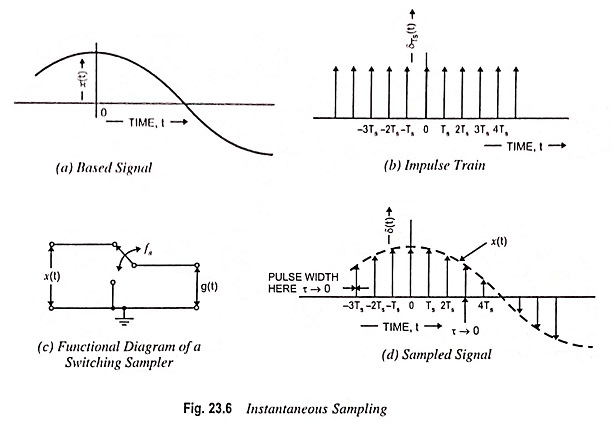The working principle of this circuit is quite easy. The circuit simply consists of a switch. Now if it is assumed that the closing time ‘t’ of the switch approaches zero, then the output g(t) of this circuit will contain only instantaneous value of the input signal x(t). Since the pulse width approaches zero, the instantaneous sampling provides a train of impulses of height equal to the instantaneous value of the input signal x(t) at the sampling instant.

The train of impulses may be represented as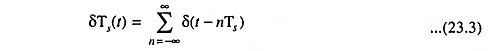This is known as sampling function and its waveform is shown in Fig. 23.6 (d).

The sampled signal g(t) is expressed as the multiplication of x(t) and δTs(t). Thus,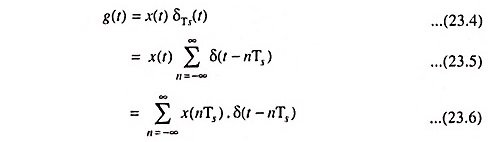The Fourier transform of the ideally sampled signal given by the above equation may be expressed as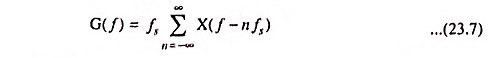The above equation gives the spectrum of ideally sampled signal. It shows that the spectrum X(f) is periodic in fs and weighted by fs. However, it may be noted that ideal or instantaneous sampling is possible only in theory, since it is impossible to have a pulse whose width approaches zero. Practically flat-top sampling and natural sampling are used.

### Natural Sampling:

In this types of sampling techniques, the pulse has a finite width equal to τ.

Let us consider an analog continuous time signal x(t) to be sampled at the rate of fsHz, fs being assumed higher than Nyquist rate such that sampling theorem is satisfied.

Again let us consider a sampling function c(t) which is a train of periodic pulses of width τ and frequency equal to fs Hz.

With the help of a natural sampler, a sampled signal g(t) is obtained by multiplication of sampling function c(t) and input x(t).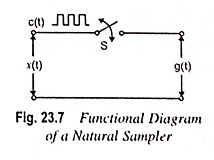Now, from Fig. 23.7, we have

When c(t) goes high the switch S is closed.

Therefore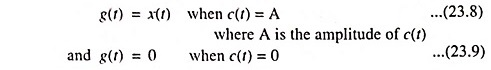The waveforms of signals x(t), c(t) and g(t) have been illustrated in Fig. 23.8 (a), (b) and (c) respectively.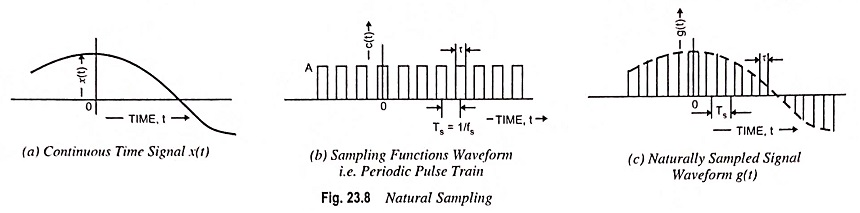The sampled signal g(t) may also be described mathematically as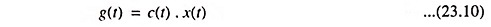Here c(t) is the periodic pulse train of width τ and frequency fs.

The sampling here is termed natural sampling, since the top of each pulse in g(t) retains the shape of its corresponding analog segment during the pulse interval.

### Flat Top Sampling or Rectangular Pulse Sampling:

Flat top sampling like natural sampling is also a practically pos­sible sampling method. But natural sampling is little complex whereas it is quite easy to get flat top samples.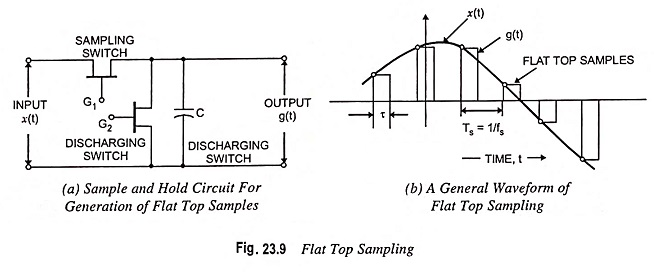In flat top sampling, the top of the samples remains constant and is equal to the instantaneous value of the baseband signal x(t) at the start of sampling. The duration or width of each sample is τ and sampling rate is equal to fs = 1/Ts. Figure 23.9 (a) shows the functional diagram of a sample and hold circuit which is used to generate the flat top samples. Figure 23.9 (b) depicts the general waveform of flat top samples.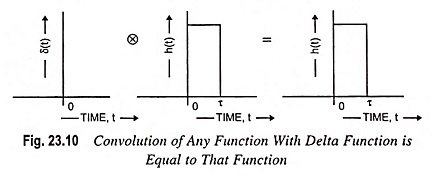From Fig. 23.9 (b), it may be noted that only starting edge of the pulse represents instantaneous value of the baseband signal x(t). Also, the flat top pulse of g(t) is mathematically equivalent to the convolution of instantaneous sample and a pulse h(t) as depicted in Fig. 23.10. This means that the width of the pulse in g(t) is determined by the width of the h(t) and the sampling instant is determined by delta function.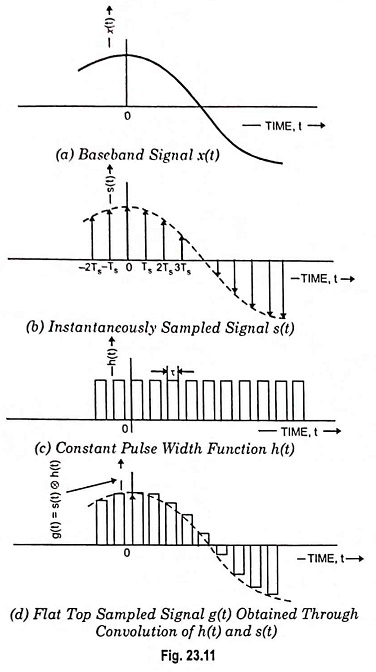In Fig. 23.9 (b), the starting edge of the pulse represents the point where baseband signal is sampled and width is determined by function h(t). Therefore output g(t) will be expressed as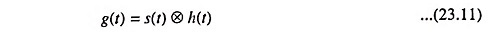The above equation has been explained in Fig. 23.11. Now, from the property of delta function, we know that for any function f(t)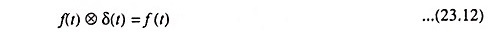This property is used to obtain flat top samples. It may be noted that to obtain flat top sampling, we are not applying the Eq. (23.12) directly here i.e., we are applying a modified form of Eq. (23.12). This modified equation is Eq. (23.11).

Thus, in this modified equation, we are taking s(t) in place of delta function δ(t). Observe that δ(t) is a constant amplitude delta function whereas s(t) is a varying amplitude train of impulses. This means that we are taking s(t) which is an instantaneously sampled signal and this is convolved with function h(t) as in Eq. (23.11).

Therefore, on convolution of s(t) and h(t), we get a pulse whose duration is equal to h(t) only but amplitude is defined by s(t).

Now we know that the train of impulses may be represented mathematically as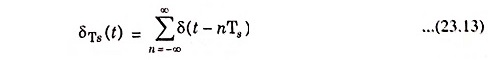The signal s(t) is obtained by multiplication of baseband signal x(t) and δTs(t). Thus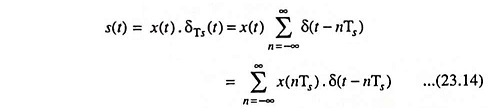Now, sampled signal g(t) is given as Eq. (23.11)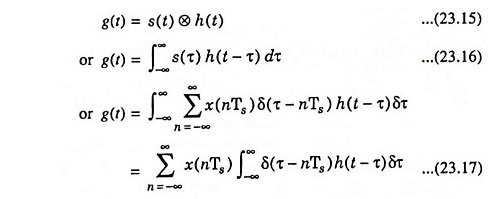According to shifting property of delta function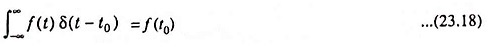Using Eqs. (23.17) and (23.18), we have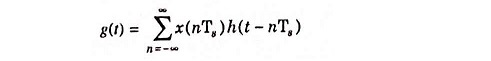The above equation represents value of g(t) in terms of sampled value x(nTs) and function h(t-nTs) for flat top sampled signal.

Now, again from Eq. (23.11), we have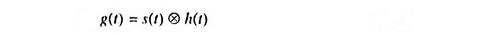Taking Fourier transform of both sides of above equation, we have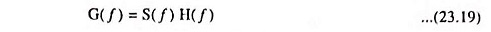and S(f) is given as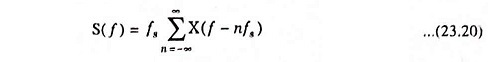So, Eq. (23.19) becomesThus spectrum of flat top sampled signal is

Scroll to Top